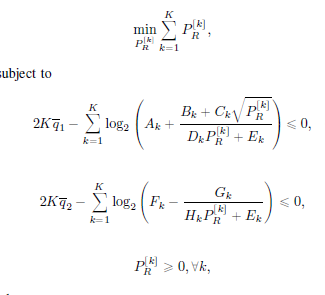# Optimisation KKT formulating into CVXI need to find PR where the rest of it (Ak,Bk,Ck,Dk,Ek,Fk,Gk,Hk, q1,K) are numbers. How to formulate these equations into CVX? Thanks

The first constraint is not convex. For instance, choosing all the input constants to be 1, then the 2nd derivative of `log(1+sqrt(p)/(p+1))` is negative for p = 2 and positive for p = 3.

Unless with the combination of all input values over the whole summation, it all nets together somehow to be a convex constraint. But certainly not convex in general.

And KKT really doesn’t come into play when trying to formulate this in CVX.